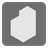Stacking Cubes
Undefined
English JA

In this mission you'll have to stack the cubes.

You are given a list of cube details (a tuple of 3 integers: X coordinate, Y coordinate, and edge length).
You have to return the maximum stacking height of the cubes.

Here are some of the conditions:
• each coordinate is the minimum value;
• all edges are parallel to the coordinate axes;
• the order of cube stacking is arbitrary, but the X and Y coordinates of the cubes can't be changed;
• if at least 1x1 faces of the cubes touch, they will be stacked.

Note:

• You don't necessarily need to use all of the cubes.

Examples :

```stacking_cubes([(0, 0, 2), (1, 1, 2), (3, 2, 2)]) == 4
stacking_cubes([(0, 0, 2), (1, 1, 2), (1, 2, 1), (2, 2, 2)]) == 6
stacking_cubes([(0, 0, 2), (0, 2, 2), (0, 2, 2), (2, 0, 2), (2, 0, 2), (2, 0, 2), (2, 0, 2)]) == 8```Input: A list of tuples of 3 integers.

Output: An integer.

Precondition:

• 2 ≤ len(cubes) ≤ 50
• -100 ≤ X, Y coordinate ≤ 100